JMSLTM Numerical Library 7.2.0
com.imsl.stat

## Class VectorAutoregression

• All Implemented Interfaces:
Serializable, Cloneable

```public class VectorAutoregression
extends Object
implements Serializable, Cloneable```
Performs vector autoregression for a multivariate time series.

This class contains methods for modeling multivariate time series of the form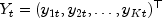where each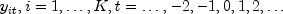is a real-valued time series indexed by t. Define the K dimensional mean process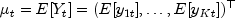and the cross-covariance matrix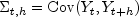where for each (t,h),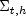is a K * K real-valued matrix whose (i,j)t,h element is the covariance between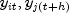: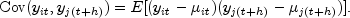The vector autoregressive model, or VAR, has a number of equivalent forms. A general form is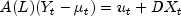where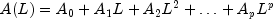is a p-th degree matrix polynomial in the lag or backshift operator, L. Note that p is the specified autoregressive lag and ut is the error process. The error process is assumed to be a K dimensional white noise series, with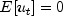and nonsingular covariance matrix,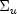, which is constant over time. The coefficient matrices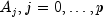are K * K matrices containing the coefficients of the model. The matrix Xt represents a matrix of deterministic terms such as trend or seasonal variables, and D represents the associated coefficient matrix of Xt.

Example, Serialized Form
• ### Constructor Summary

Constructors
Constructor and Description
`VectorAutoregression(TimeSeries ts)`
Constructor for the class.
• ### Method Summary

Methods
Modifier and Type Method and Description
`double[]` `getARConstants()`
Returns the current settings of the constants used in the autoregression model.
`int[]` `getARModel()`
Returns the autoregressive model configuration.
`double[][]` `getEstimates()`
Returns the parameter estimates (coefficients) of the vector autoregression model.
`double[][]` `getForecasts()`
Returns the h-step ahead forecast at times t=nT, nT+1, ..., T, where h=1,2, ..., `maxStepsAhead`.
`boolean` `isA0Flag()`
Returns the state of `A0Flag`.
`void` `setA0Flag(boolean A0Flag)`
Sets the flag to include the leading autoregressive coefficient matrix in the model.
`void` `setARConstants(double[] arConstants)`
Sets the constants for the autoregressive model.
`void` `setARLag(int arLag)`
Sets the autoregressive lag parameter.
`void` `setARModel(int[] arModel)`
Sets the form of the autoregressive terms of the model.
`void` `setCenter(boolean center)`
Sets the flag to center the data.
`void` `setMaxLag(int maxLag)`
Sets the maximum lag.
`void` `setScale(boolean scale)`
Sets the flag to scale the data.
`void` `setTrend(boolean trend)`
Sets the flag to fit a trend parameter in the model.
• ### Methods inherited from class java.lang.Object

`clone, equals, finalize, getClass, hashCode, notify, notifyAll, toString, wait, wait, wait`
• ### Constructor Detail

• #### VectorAutoregression

`public VectorAutoregression(TimeSeries ts)`
Constructor for the class.
Parameters:
`ts` - a `TimeSeries` object.
• ### Method Detail

• #### getARConstants

`public double[] getARConstants()`
Returns the current settings of the constants used in the autoregression model.
Returns:
a `double` array containing the constants used in the autoregression model. If the value is `null`, the default values are active.
• #### getARModel

`public int[] getARModel()`
Returns the autoregressive model configuration.
Returns:
an `int` array specifying the autoregressive configuration. If `null`, then all parameters are active in the current model.
• #### getEstimates

`public double[][] getEstimates()`
Returns the parameter estimates (coefficients) of the vector autoregression model.
Returns:
a `double` array containing the estimated parameters (coefficients).
• #### getForecasts

`public double[][] getForecasts()`
Returns the h-step ahead forecast at times t=nT, nT+1, ..., T, where h=1,2, ..., `maxStepsAhead`.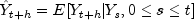uses the conditional expectation of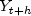given the historical information known at time t. The estimated VAR model approximates the conditional expectation and the forecasts are generated from the estimated VAR model via the following recursion: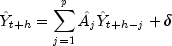where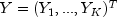is the vector time series and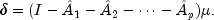Returns:
a `double` matrix T-nT by `maxStepsAhead` * K containing the forecasts.

Note that the forecast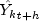is stored in location [t-nT][(h-1)*K + k] of the returned forecast matrix.

• #### isA0Flag

`public boolean isA0Flag()`
Returns the state of `A0Flag`.
Returns:
a `boolean` which specifies whether or not the leading coefficient matrix is nontrivial.
• #### setA0Flag

`public void setA0Flag(boolean A0Flag)`
Sets the flag to include the leading autoregressive coefficient matrix in the model.

When `true`, a nontrivial, lower-triangular leading autoregressive coefficient matrix, A0, will be estimated in the model. When `false`, A0 is the constant identity matrix, which is also the default case.

Parameters:
`A0Flag` - `boolean` indicating whether to fit the leading coefficient matrix.

Default: `A0Flag`=`false`.

• #### setARConstants

`public void setARConstants(double[] arConstants)`
Sets the constants for the autoregressive model. See discussion in `setARModel(int[])` for details.
Parameters:
`arConstants` - a `double` array specifying the autoregressive model constants.

The input array `arConstants` must be of length (`A0Flag`+`arLag`)*K*K, such that for indices 1=0,...,(`A0Flag` + `arLag`), i=0,...,K-1, and j=0,...,K-1, `arConstants[`l*K*K +i*K + j`]` specifies a constant value for parameter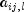.

Default: `arConstants[`i`]`=0.
• #### setARLag

`public void setARLag(int arLag)`
Sets the autoregressive lag parameter. It must be nonnegative and less than `maxLag`.
Parameters:
`arLag` - an `int` specifying the desired lag for the autoregressive terms. Default: `arLag`=1.
• #### setARModel

`public void setARModel(int[] arModel)`
Sets the form of the autoregressive terms of the model.

Without loss of generality, assume that the series mean is 0 and that there are no deterministic terms. Then we can write the vector autoregression model of lag p as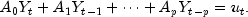Each of the matrices Aj represents K * K unknown coefficients. In practice, many of the (p+ 1) * K * K coefficients are restricted to be 0 or otherwise constant. The leading coefficient matrix A0 must be lower-triangular and is very often equal to the identity matrix (this is the default behavior). Fully expanded the model looks like K equations in K(p+1) unknowns.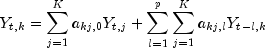Consider each coefficient matrix as the vector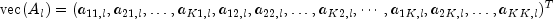for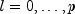.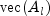is just the columns of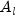appended to each other. To specify a configuration different from the default, append the vectorized coefficient matrices as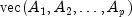or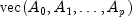when including a nontrivial leading coefficient matrix. Set the value at index l*K*K + j*K + i to 1 or -1 to fitor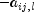in the model. Set the value to 0 to excludeas a parameter. Note that for the leading coefficient matrix,l= 0, the value at j*K + i is ignored unless i is at least j, i.e., only the lower triangle of A0 can be nontrivial.

Parameters:
`arModel` - an `int` array specifying the autoregressive model parameters.

For indices l=0,...,(`A0Flag`+`arLag`), i=0,...,K-1, and j=0,...,K-1, `arModel[`l*K*K +i*K + j`]` = {-1,1} indicates that the coefficient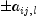is a parameter (to be estimated) in the model.

Default: A0 is the identity matrix and `arModel[`i`]`=1 for all i.

• #### setCenter

`public void setCenter(boolean center)`
Sets the flag to center the data. If `center`=`true`, column means are subtracted from the data.
Parameters:
`center` - a `boolean` indicating whether or not column means should be subtracted from the data.

Default: `center`=`false`.

• #### setMaxLag

`public void setMaxLag(int maxLag)`
Sets the maximum lag.
Parameters:
`maxLag` - an `int` specifying the maximum lag to consider in the first (pure VAR) stage regression.
• #### setScale

`public void setScale(boolean scale)`
Sets the flag to scale the data.

If `scale`=`true`, the data values are mean-centered and then divided by the standard deviation.

Parameters:
`scale` - a `boolean`

Default: `scale`=`false`.

• #### setTrend

`public void setTrend(boolean trend)`
Sets the flag to fit a trend parameter in the model. Set to `true` to include a deterministic trend in the model.
Parameters:
`trend` - a `boolean` specifying whether or not to include a deterministic trend.

Default: `trend`=`false`.

JMSLTM Numerical Library 7.2.0